## Binary Sequences

2.1 A variety of abstractions built upon binary sequences can be used to represent all digital data.
2.1.2 Explain how binary sequences are used to represent digital data. [P5]
2.1.2A A finite representation is used to model the infinite mathematical concept of a number.
2.1.2B In many programming languages, the fixed number of bits used to represent characters or integers limits the range of integer values and mathematical operations; this limitation can result in overflow or other errors. Exclusion Statement (2.1.2B): Range limitations of any one language, compiler, or architecture are beyond the scope of this course and the AP Exam.
2.1.2C In many programming languages, the fixed number of bits used to represent real numbers (as floating point numbers) limits the range of floating point values and mathematical operations; this limitation can result in round off errors.
2.1.2D The interpretation of a binary sequence depends on how it is used.
2.1.2E A sequence of bits may represent instructions or data.
2.1.2F A sequence of bits may represent different types of data in different contexts.
6.2.2K The latency of a system is the time elapsed between the transmission and the receipt of a request.
6.2.2J The bandwidth of a system is a measure of bit rate – the amount of data (measured in bits) that can be sent in a fixed amount of time.

All data is stored in sequences of binary (ones and zeros), and how any binary sequence is interpreted depends on how it will be used. Binary sequences are used to represent instructions to the computer and various types of data depending on the context.

Computers store information in binary using bits and bytes. A bit is a "0" or "1". A byte is eight bits grouped together like 10001001.

Bytes have 8 places, so the left-most place is 27 (or 128). Practice what you've learned: What is 100010012 in base 10?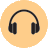• bit – a single unit of data that can only have one of two values (such as a 1 or 0)
• bit rate – the amount of data (measured in bits) that can be sent in a specific amount of time
• bandwidth – the transmission capacity of a system (measured by bit rate)
• latency – time between the transmission and the receipt of a message

As computers become more powerful, they are capable of handling more information. We used to measure computer memory in kilobytes; one kilobyte is 210 (1,024) bytes, which is about 103, so we call it a "kilo". These days, your computer memory is likely to be measured in megabytes. One megabyte is 220 (about 1 million) bytes.

Your hard drive space is likely to be measured in gigabytes, terabytes, or even petabytes. One gigabyte is 230 (about 1 billion) bytes, one terabyte is 240 (about 1 trillion) bytes, and one petabyte is 250 (about 1 quadrillion) bytes.

 measure amount example bit either a 1 or a 0 1 byte 8 bits 11011001 kilobyte 210 (1,024) bytes a couple of paragraphs megabyte 220 (1,048,576) bytes about 1 book gigabyte 230 (1,073,741,824) bytes a little more than 1 CD terabyte 240 (1,099,511,627,776) bytes about 1,500 CDs petabyte 250 (1,125,899,906,842,624) bytes about 20 million filing cabinets of text

### Storing Integers

Depending on the programming language used, an integer might be stored with two or perhaps four bytes of data allowing for a range of about -32,768 to 32,767 (with two bytes) or -2,147,483,648 to 2,147,483,647 (with four bytes).

#### Why are these the ranges for integers?

Two bytes is 16 bits, so two bytes can represent 216 = 65,536 different values. We use about half of these to represent negative numbers, about half for postive numbers, and one to represent zero.

Four bytes is 32 bits, so four bytes can represent 232 = 4,294,967,296 different values. Again, we use about half for negative numbers, half for postives, and one for zero.

What about bigger numbers? Whatever the specific size is for storing integers (two bytes, four bytes, etc.), it will limit the range of the integer values that can be stored and the mathematical operations that can be performed. Values that exceed this limitation may result in errors such as overflow errors because big values overflow the amount of space allocated to store one number.
1. You do not need to learn the factorial function for this class (we are just using it to discuss big numbers), but you will probably see it again in a math class.
The factorial of a positive integer n (written "n!") is the product of all the integers from 1 to n. For example:
5! = 1 \times 2 \times 3 \times 4 \times 5 = 120 Load this project, and try out these inputs:
You might see different results based on your computer processor.
1.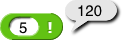2.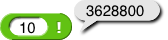3.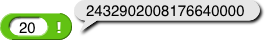2. Then, try 200!.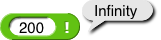What's going on? Although 200! is very large, it's not infinite. This report is the result of the size limitation. If the result of a computation is bigger than than the range of numbers that can be stored, then the computer returns a special code or an overflow error.

In Snap!, there are special codes for infinity, –infinity (smaller than any finite value), and "Not a Number," which is the notification used for illegal computations such as \frac00.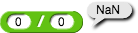#### Storing Text

You'll learn more about Unicode if you do the Caesar Cipher Project in the Cybersecurity lab, where you'll create a program to encrypt a message.

It's common for programming languages to use a specific number of bits to represent Unicode characters or integers. Unicode is a system that allows computers to store letters as integers. For example, capital A is Unicode number 65, which is 010000012.

### Floating Point

Many languages have another data type used to represent real numbers (including decimals and numbers too big to be stored as integers) called floating point, which is essentially a binary version of scientific notation. It's called floating point because the decimal point "floats" to just after the first digit.

1. Try 30!.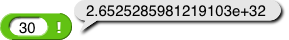That e+32 is just a different way to write scientific notation. The "e+" means "times ten to the power of" so: 2.6525285981219103\text{e}+32 = 2.6525285981219103\times10^{32} =265,252,859,812,191,030,000,000,000,000,000.

Floating point allows computers to store very large numbers and also decimals, but the format still has a specific number of bits, and that limits the range of floating point values and mathematical operations just like with integers. However with floating point, values that exceed the limitation may result in round off errors instead.

Most real numbers can't be represented exactly—even with floating point.

1. For example, try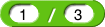.

The decimal representation of \frac13 is 0.33333... It has infinitely many digits, so the closest you can come in floating point isn't exactly \frac13; it gets cut off after a while.

Roundoff errors can result in some pretty weird calculations...

1. Try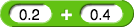and then try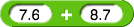.

This isn't a bug with Snap!. Many other languages (like JavaScript) will report the same values for these calculations.

Computer arithmetic on integers is straightforward. Either you get an exactly correct integer result or, if the result won't fit in integer representation, you get an overflow error and the result is, usually, converted to floating point representation (like 30! was).

By contrast, computer arithmetic on floating point numbers is hard to get exactly right. Prior to 1985, every model of computer had a slightly different floating point format, and all of them got wrong answers to certain problems. This situation was resolved by the IEEE 754 floating point standard, which is now used by every computer manufacturer and has been improved several times since it was created in 1985.

How does a programming language know whether to interpret a bit sequence as an integer, a floating point, a text string of Unicode characters, an instruction, or something else? Programming languages differ in how they do this, but there's always some extra bit sequence that encodes the data type of any sequence of bits that tells the computer how to interpret it.

At the lowest level of software abstraction, everything in a computer is represented as a binary sequence. For example:
• A Boolean value is a single bit, 0 for false and 1 for true.
• A text string is a sequence of Unicode character codes, each of which is stored as a separate integer.
• Lists and blocks are binary sequences too.

1.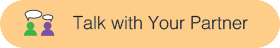What's the rightmost digit in the binary representation of 15551212?
2. What's the rightmost bit ("binary digit") of 123456789?
3. Develop a rule for finding the rightmost bit of any base 10 number.